Electrochemical series What can it tell us? The electrochemical series, shown on the right, is a list of half cells arranged in order of their increasing electrode potential values. Oxidants are placed on the left and their conjugate reductants are placed on the right. The electrode potential of a half cell is the voltage produced when it is coupled to the hydrogen half cell, shown in red in the electrochemical series on the right, under standard conditions. Standard conditions are 1.00M solutions, 1 atm pressure and 25 oC. An electrochemical series, written in this way, can be a very useful tool in electrochemistry. But, what can it reveal? 1) It can predict if a reaction will take place spontaneously. If the oxidant is above the reductant in the electrochemical series then a spontaneous reaction is likely to occur. For example if magnesium metal is placed in a solution of Cu2+ ions then a spontaneous reaction is predicted to take place. That is because the oxidant, Cu2+, is above Mg(s) on the series. What the electrochemical series will not do is predict how fast a reaction will take place. 2) It also arranges the metals in increasing order of reactivity. The lower a metal appears on the table the greater its ability to donate electrons, in other words the greater its reactivity. Lithium is at the bottom indicating that it is a very reactive metal. 3) We can use the series to predict the theoretical voltage that can be produced by a galvanic cell, given that the galvanic cell is operating under standard conditions and is formed by two half cells that appear in the table. For example , consider the galvanic cell formed by a zinc and copper half cells. To work out the voltage, take the Eo value of the oxidant and subtract the Eo value of the reductant. In this case the galvanic cell should produce (+0.34 - -0.76) 1.10V The further apart the oxidant is from the reductant on the series, the greater the voltage produced. 4) Oxidation and reduction half reactions can also be obtained from the electrochemical series. In order to do this we follow two simple steps. Lets see an example. Write the oxidation and reduction half equations for the reaction that may or may not occur if an iron nail is placed in a solution of Ag+ ions. Step 1 Will a spontaneous reaction occur?  If yes proceed to step 2 Step 2 -    identify the oxidant and write the half equation as it is shown on the electrochemical series. This is the reduction half equation -    identify the reductant and write the half equation in the backward direction as that shown on the electrochemical series. This is the oxidation half equation.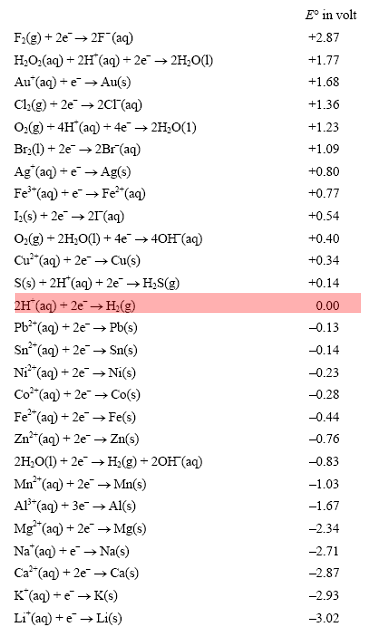Try the following exercises. 1. Predict the voltage of the following galvanic cells using the electrochemical series shown above. Solution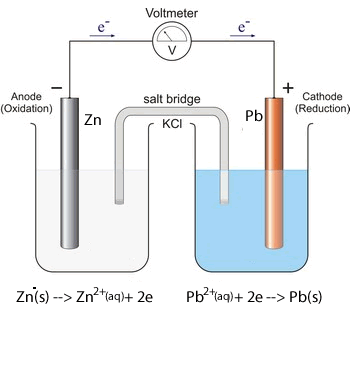Solution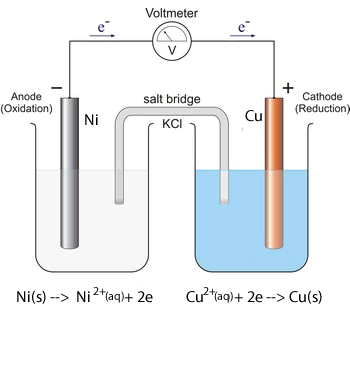Solution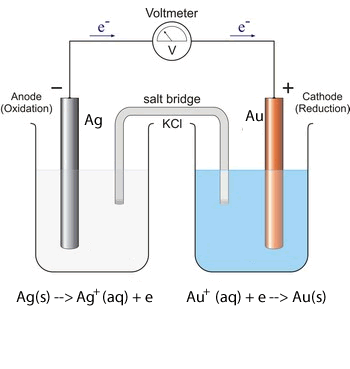Solution Electrochemical series2. Predict if a spontaneous reaction will occur in the following circumstances. i. Pb metal dipped in AgNO3 solution. Solution ii. Cobalt metal dipped in liquid bromine (Br2). Solution iii. Calcium metal placed in pure water. Solution iv. Gold metal placed in Mg(NO3)2 solution. Solution3. Which of the following chemical reactions are spontaneous? a. Use the electrochemical series to write the oxidation and reduction half equations for each spontaneous reaction and draw a viable, labelled, galvanic cell for each spontaneous reaction.      i.   2Al(s) + 3Pb2+(aq)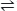2Al 3+(aq) + 3Pb(s)           Solution             Galvanic cell    ii.  4OH-(aq) + 2Co2+(aq)O2(g) + 2H2O(l) + 2Co(s)          Solution            Galvanic cell   iii.   2Fe3+(aq) + Sn(s)Sn2+(aq) + 2Fe2+(aq)         Solution           Galvanic cell   iv.   Cu(s) + 2H+(aq)H2(g) + Cu2+(aq)         Solution          Galvanic cell    v.  Ni(s) + 2Ag+(aq)2Ag(s) + Ni2+(aq)        Solution         Galvanic cell